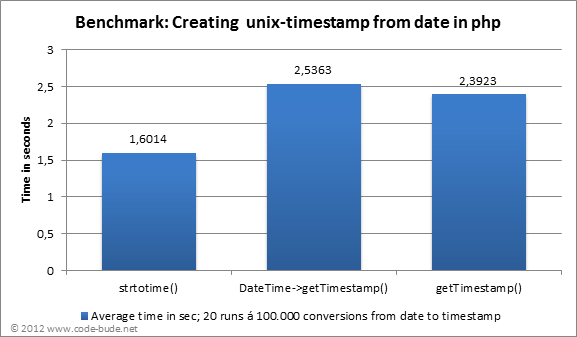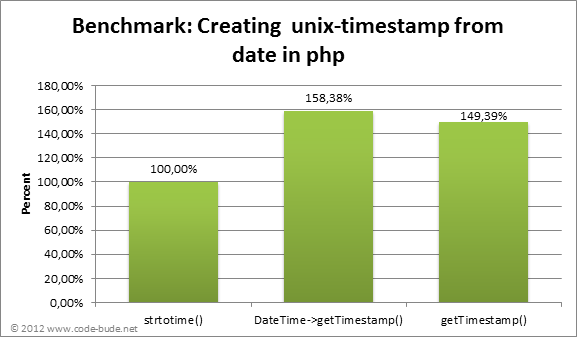# strtotime() vs DateTime vs getTimestamp in PHP

In the article, presented two approaches. Firstly, using the strtotime() method and another with the DateTime class.

It was pointed out that the strtotime() variant is probably faster. Because I wasn’t sure about this, I decided to make a small performance test and share the results with you in this article.

How did I test the performance?

Since the question aims on strtotime() vs. DateTime, the DateTime class is contrary to the strtotime() function. So because DateTime class offers more functionality, I had first to create the DateTime object and then get the timestamp of it, because the strtotime function gives a timestamp as return value and I wanted to compare the time needed to generate the identical output.

As input I have taken a date without a custom time – say a year, month and day indication. In addition, I tested a third function. What exactly is going on with the getTimestamp () function, I will explain later in the article. The three function calls for my following benchmark look as follows:

 1 2 3 4 5 6 7 8 9 `//strtotime()` `\$a``=``strtotime``(``"2012-05-28"``);` `//DateTime` `\$date``=``new` `DateTime(``"2012-05-28"``);` `\$a` `= ``\$date``->getTimestamp();` `//getTimestamp()` `\$a` `= getTimestamp(``"2012-05-28"``);`

How did I measure?

As a reference value I wanted to specify the time in seconds. To measure time, I used the following functions:

 1 2 3 4 5 6 7 8 9 10 11 `//Time prior to the execution of a variant` `\$startTime` `= microtime(true);` `/***************************` `  ``Run variant / tests` `****************************/` `//Time after the end of the test` `\$endTime` `= microtime(true);` `//Period of the test` `\$elapsedTime` `= ``abs``(``\$startTime` `- ``\$endTime``);`

Each of the functions has been called in a loop for 100000 times while the time was measured. To compensate for fluctuations and measurement inaccuracies, the measurement for each variant was performed 20 times and the mean/average “elapsed time” of 20 measurements was taken. Finally the code for my benchmark looked as follows:

 1 2 3 4 5 6 7 8 9 10 11 12 `\$total` `= 0;` `for` `(``\$runs` `= 0; ``\$runs` `< 20; ``\$runs``++)` `{` `    ``\$startTime` `= microtime(true);` `    ``for` `(``\$i` `= 0; ``\$i` `< 100000; ``\$i``++)` `    ``{` `        ``//Execute function / variant` `    ``}` `    ``\$endTime` `= microtime(true);` `    ``\$total` `+= ``abs``(``\$startTime` `- ``\$endTime``);` `}` `\$elapsedTime` `= sprintf(``"%.4f sec."``, (``\$total``/20));`

I also have added the following line of code at the beginning of the benchmark scripts to set the correct time zone for the strtotime()-function as well as for the DateTime variant.

 1 `date_default_timezone_set(``"Europe/Berlin"``);`

What is the result?

The result ended up so as it had already suspected by Christoph. The DateTime variant was behind the strtotime() function. Second place went to the getTimestamp () function. In initial tests, this cut off so good that made it to the first place. Here, however, still lacked some calculations, which have finally catapulted getTimestamp() to second place.

I have summarized the whole thing in two small graphics.What’s up with the getTimestamp()?

The getTimestamp () function is not an official PHP function, but a small in-house development from me. After doing a few tests with strtotime() – which had out performed the DateTime version, I wanted to see if I myself can create a faster version.

As can be seen from the above graphs, mine function was not quite successful. In initial tests it had indeed worked, but I was made aware in the comments that my getTimestamp() function would not always return the correct values.  After I have added the missing switching between summer and winter time (for our room – in America will be converted on other dates!), my function is now a little bit slower than the strtotime() function.

My getTimestamp function looks like this:

 1 2 3 4 5 6 7 8 9 10 11 12 13 14 15 16 17 18 19 20 21 22 23 24 25 26 27 28 29 30 31 32 33 34 35 36 37 38 39 40 41 42 43 44 45 46 `function` `getTimestamp(``\$timeStr``)` `{` `    ``\$daysInMonth` `= ``array``(0,31,59,90,120,151,181,212,243,273,304,334);` `    ``\$dateArr` `= ``explode``(``"-"``, ``\$timeStr``);` `    ``//Add years to the day of the year entered` `    ``\$days` `= (``\$dateArr``-1970) * 365;` `    ``//Add leap years, days` `    ``for` `(``\$i` `= 1972; ``\$i` `<= ``\$dateArr``; ``\$i``+=4)` `    ``{` `        ``if` `((``\$i``%4==0 && ``\$i``%100 != 0) || ``\$i``%400==0)` `            ``\$days``++;` `    ``}` `    ``//Days until 31.03. of the year` `    ``\$daysTimeswitch` `= ``\$days` `+ 90;` `    ``//Determine day of the week using MOD 3 . (+3 because 01.01.1970 = Thursday)` `    ``//Pull off day of the week from 31. = last Sunday of the month => Daylight saving time` `    ``\$daySummertime` `= 31 - (((``\$daysTimeswitch``%7) + 3)%7);` `    ``\$daysTimeswitch` `= ``\$days` `+ 304;` `    ``\$dayWintertime` `= 31 - (((``\$daysTimeswitch``%7) + 3)%7);` `    ``if` `((``\$dateArr`` > 3 && ``\$dateArr`` < 10)` `        ``|| (``\$dateArr`` == 3 && ``\$dateArr`` > ``\$daySummertime``)` `        ``|| (``\$dateArr`` == 10 && ``\$dateArr`` <= ``\$dayWintertime``))` `    ``{` `         ``\$summerWinterTime` `= 3600;` `    ``}` `    ``else``{` `         ``\$summerWinterTime` `= 0;` `    ``}` `    ``//Pull off leap year day` `    ``\$days` `-= ((``\$dateArr``%4==0 &&  ``\$dateArr``%100 != 0) ||  ``\$dateArr``%400==0) ? (``\$dateArr`` > 2) ? 0 : 1 : 0;` `    ``//Add days to months of the current year` `    ``\$days` `+= ``\$daysInMonth``[``\$dateArr``-1];` `    ``//Add days of the current year` `    ``\$days` `+= ``\$dateArr`` - 1;` `    ``//Pull off 3600 seconds for time shift. (Unix timestamp / UTC)` `    ``return` `\$days` `* 86400 - 3600 - ``\$summerWinterTime``;` `}`

Anyone who has attentive read the source code of the above function, perhaps wants to scream “Hold on …”. Yes, correctly I have to admit that my function gives correct values only under certain circumstances.  Following requirements must be met:

• The date must be passed in the form YYYY-MM-DD
• The date should not be older than the 01/01/1970

The strtotime() function takes also data in other formats and can calculate to a certain extent also timestamps of data before 01/01/1970. Here my function is clearly at a disadvantage and you might be wondering whether the comparison then may be unjustified. However, one must also note that the DateTime class in turn provides greater functionality than the strtotime() function. So this would also be unjustified…

And since in the benchmark it only was about the generation of timestamps from a given date, I think that my function has its right to exist. To be a stickler for details – the Unix timestamp begins on 01/01/1970 and thus my function meets the basic requirements for calculating a Unix timestamp.

Conclusion

Claiming strtotime() is faster than DateTime could be assigned by the test. In addition, I was able to determine that I’m not able to write a faster function than strtotime(). At the beginning of the tests, I thought that if I would limit myself to the minimum, I could write a faster function. Finally, the strtotime() function has a few more  options than my getTimestamp() function and however, it did not succeed.

Ultimately, the choice of method should also always be taken in the context of the project. What format is you input data? Do I perhaps like to calculate more than just the timestamp?

Finally, a question to you – the readers: Have any of you ever tried to write a function that is faster than strtotime()? And if so, which way did you go and what problems did you encounter?

Posted in Web